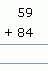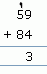Email us to get an instant 20% discount on highly effective K-12 Math & English kwizNET Programs!

#### Online Quiz (WorksheetABCD)

Questions Per Quiz = 2 4 6 8 10

Adding double digit - without carryover
Method:
1. Add the numbers in the units place.
2. Add the numbers in the tens place.
Example:Add the numbers in the units place = 3+5 = 8
Add the numbers in the tens place = 6+7 = 13Adding double digit - with carryover
Method:
1. Add the numbers in the units place.
If you get one digit, then place it on the units place.
If you get two digit number, place the units digit in the units place and carry over the tens digit.
2. Add the numbers in the tens place.
Example:

 Question Step 1 Step 2Step 1: Add the numbers in the units place = 9+4 = 13. Put 3 in the units place and carry over 1
Step 2: Add the numbers in the tens place with the carry over = 5+8+1 = 14

Directions: Add the following numbers. Also write at least ten examples of your own.
 Question 1: 57 + 57

 Question 2: 21 + 2

 Question 3: 52 + 98

Question 4: This question is available to subscribers only!

Question 5: This question is available to subscribers only!

Question 6: This question is available to subscribers only!

Question 7: This question is available to subscribers only!

Question 8: This question is available to subscribers only!